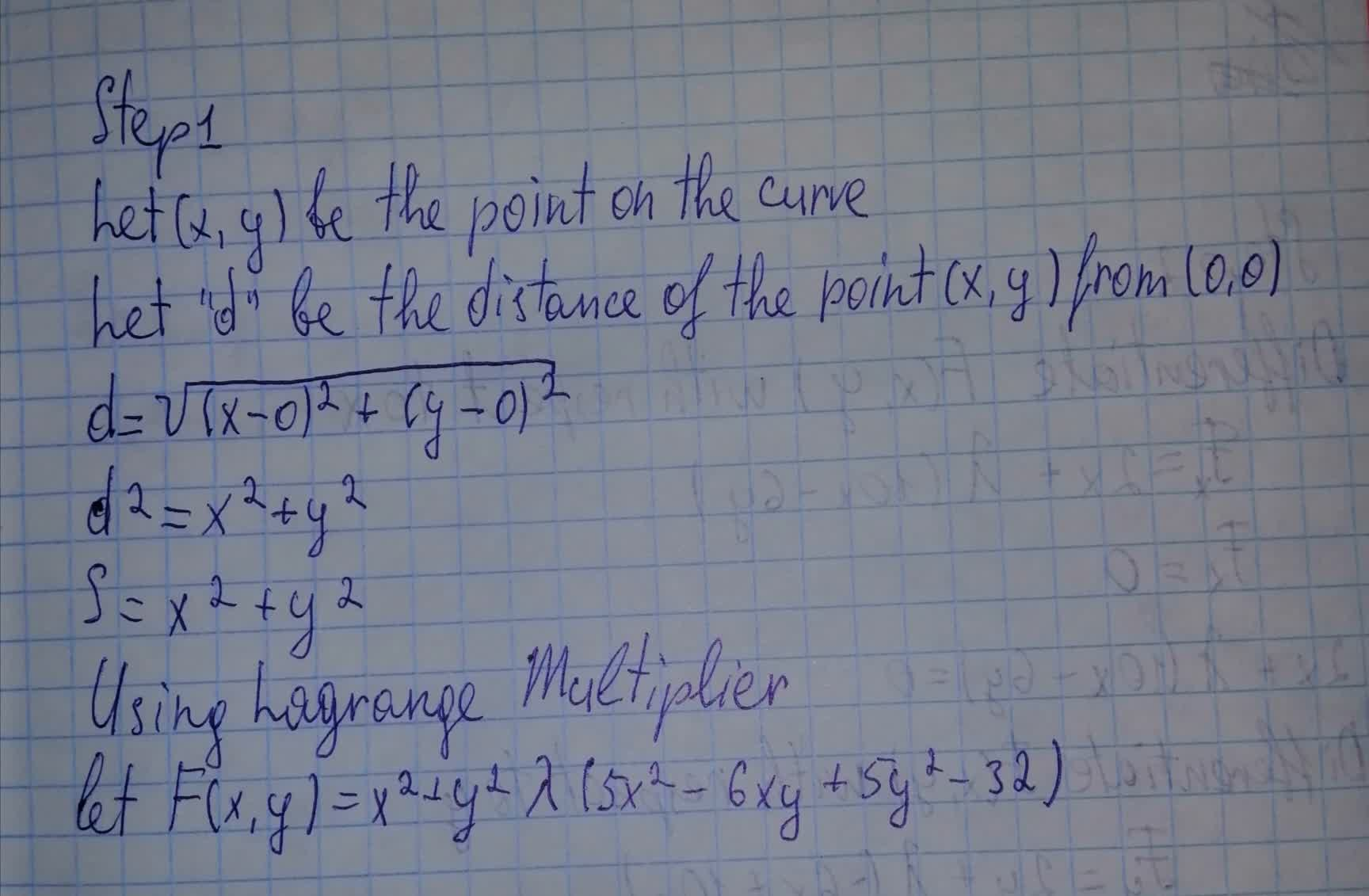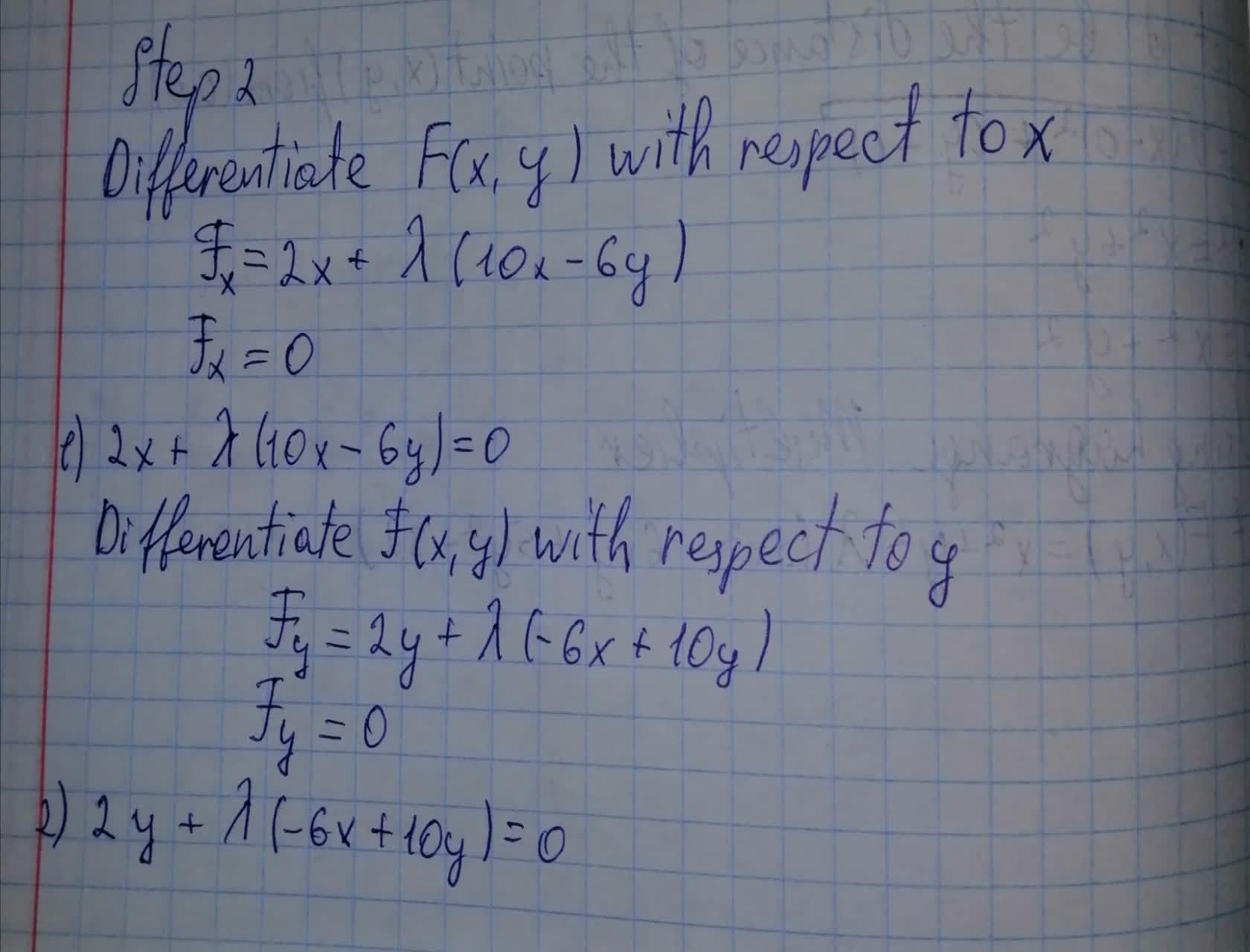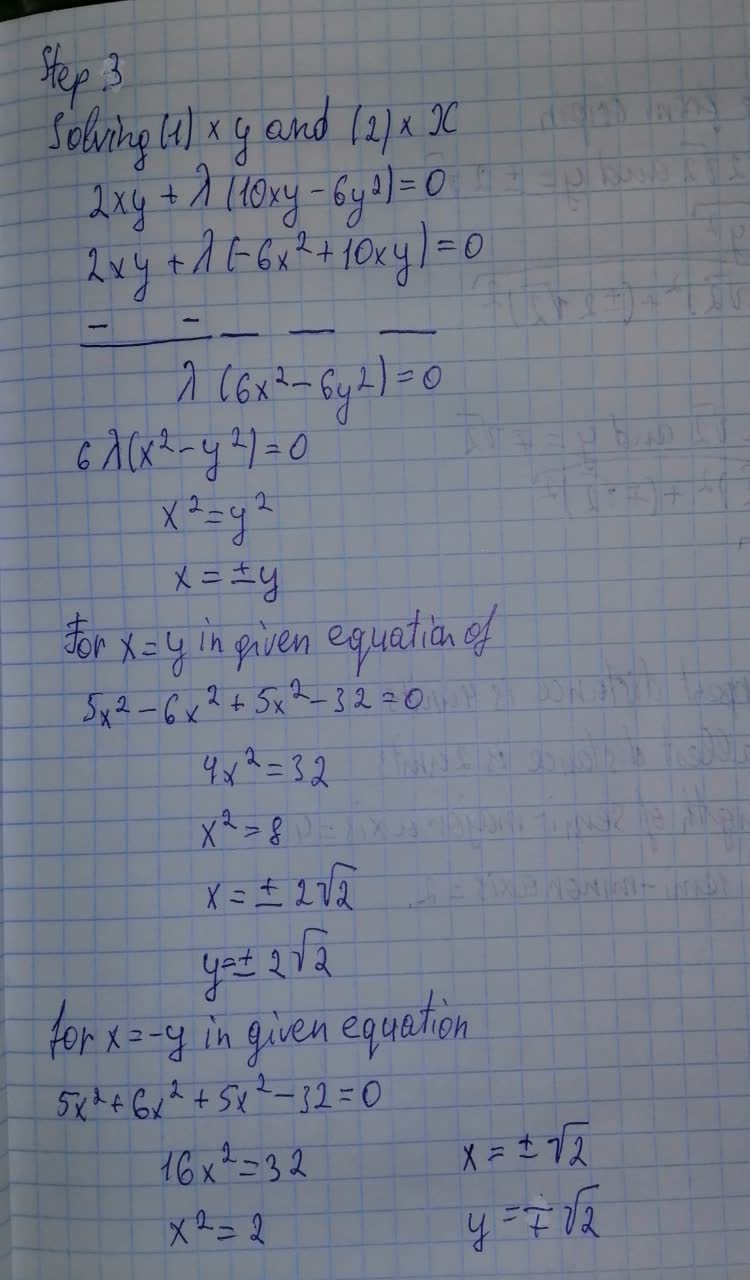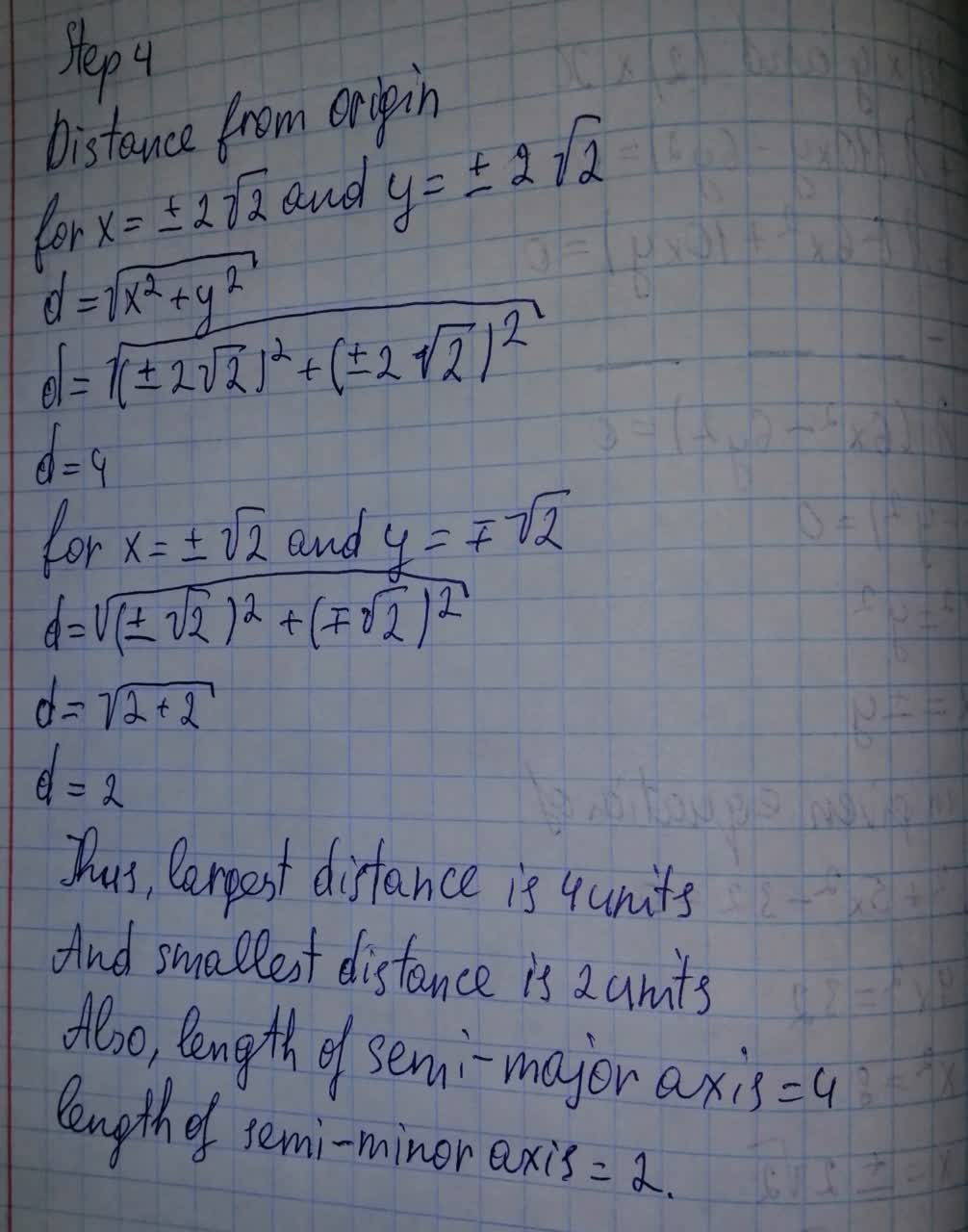# Find the largest and smallest distances from the origin to the conic whose equation is 5x^2 - 6xy + 5y^2 - 32 = 0ringearV 2021-08-08 Answered
Find the largest and smallest distances from the origin to the conic whose equation is $5{x}^{2}-6xy+5{y}^{2}-32=0$ and hence determine the lengths of the semiaxes of this conic.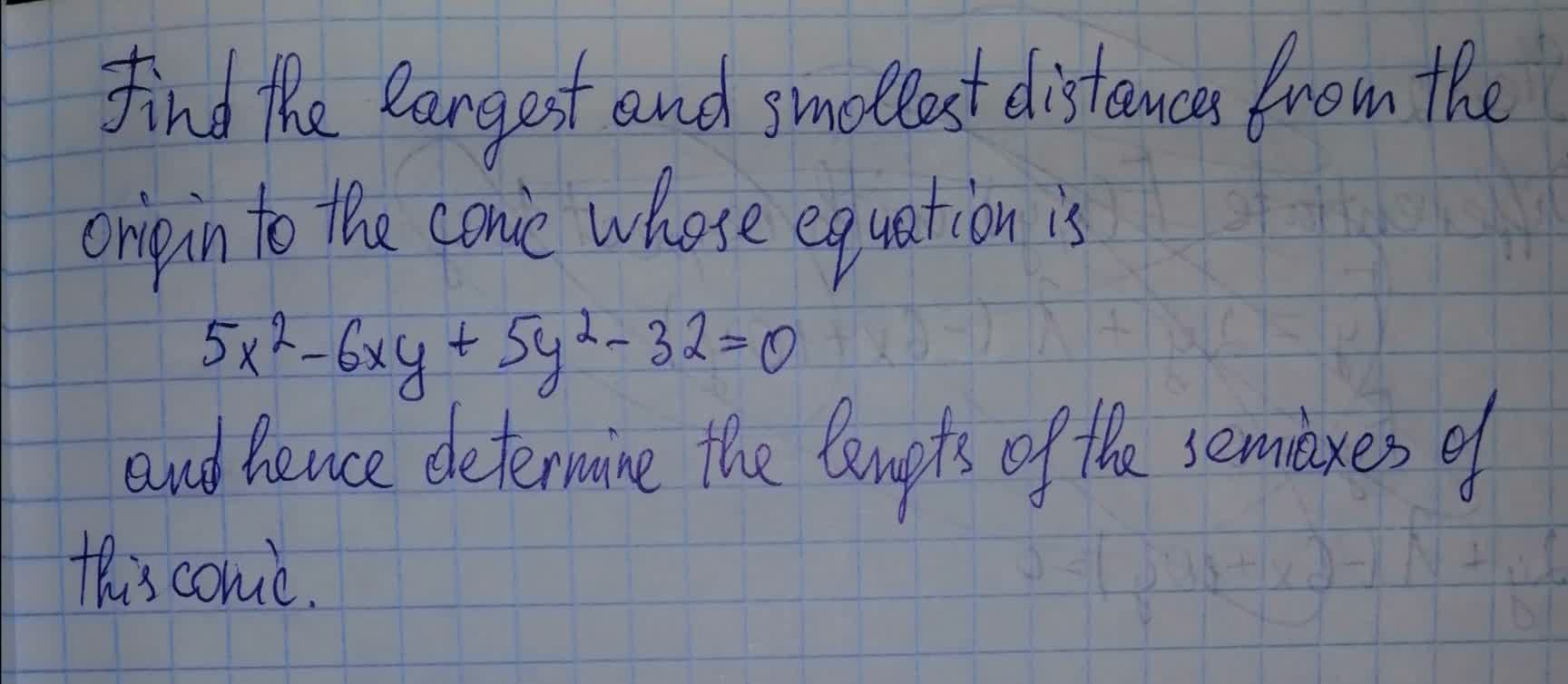You can still ask an expert for help

• Questions are typically answered in as fast as 30 minutes

Solve your problem for the price of one coffee

• Math expert for every subject
• Pay only if we can solve itMayme

Largest and smallest distances will be